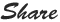# PHP For Loop

For loop:  loop is basically used to perform different operations again and again till the end condition.

Syntax:

for (Starting counter; Verifying counter; increasing counter)
{
}

Starting counter: you can declare variable and assign initial counter for loop
Verifying stop counter: While running it will verify where to stop the execution of for loop.
Increasing counter: This counter will increase its value till the verifying stop counter value

In below example 1:

"\$a=1" is starting counter
"\$<=10" is verifying stop counter that means it will verify the condition until it reaches to stop counter i.e. 10 is stop counter here.
\$a++ is the Increasing counter, it will increment till stop counter 10, the double plus sign represent the increase counter by 1
You can also write like this "\$a+2" if you want to increase counter by 2.

Example 1: Increase Loop

<?php

for(\$a = 1; \$a<=10 ; \$a++)
{
echo "This is table of one : \$a <br>";
}

?>

Output:

This is table of one :1
This is table of one :2
This is table of one :3
This is table of one :4
This is table of one :5
This is table of one :6
This is table of one :7
This is table of one :8
This is table of one :9
This is table of one :10

Example 2: Decrease Loop (Reverse Loop)

<?php

for(\$a = 10; \$a<=1 ; \$a--)
{
echo "This is Reverse table of one : \$a <br>";
}

?>

Output:

This is Reverse table of one :10
This is Reverse table of one :9
This is Reverse table of one :8
This is Reverse table of one :7
This is Reverse table of one :6
This is Reverse table of one :5
This is Reverse table of one :4
This is Reverse table of one :3
This is Reverse table of one :2
This is Reverse table of one :1